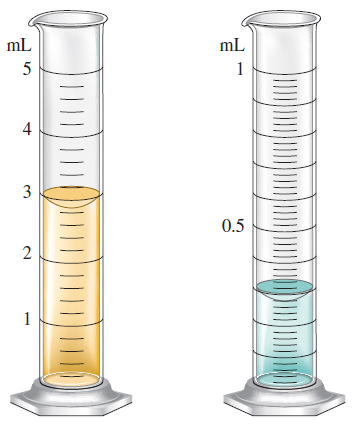# Problem: You have water in each graduated cylinder shown:You then add both samples to a beaker. How would you write the number describing the total volume? What limits the precision of this number?

###### FREE Expert Solution

addition least number of decimals

cylinder 1 = 3.80 mL

cylinder 2 = 0.280 mL

95% (379 ratings)###### Problem Details

You have water in each graduated cylinder shown:You then add both samples to a beaker. How would you write the number describing the total volume? What limits the precision of this number?

Frequently Asked Questions

What scientific concept do you need to know in order to solve this problem?

Our tutors have indicated that to solve this problem you will need to apply the Accuracy & Precision concept. If you need more Accuracy & Precision practice, you can also practice Accuracy & Precision practice problems.

What professor is this problem relevant for?

Based on our data, we think this problem is relevant for Professor Hummel's class at UIUC.

What textbook is this problem found in?

Our data indicates that this problem or a close variation was asked in Chemistry: An Atoms First Approach - Zumdahl Atoms 1st 2nd Edition. You can also practice Chemistry: An Atoms First Approach - Zumdahl Atoms 1st 2nd Edition practice problems.